• # 概率论与数理统计

## HW1

### T1.2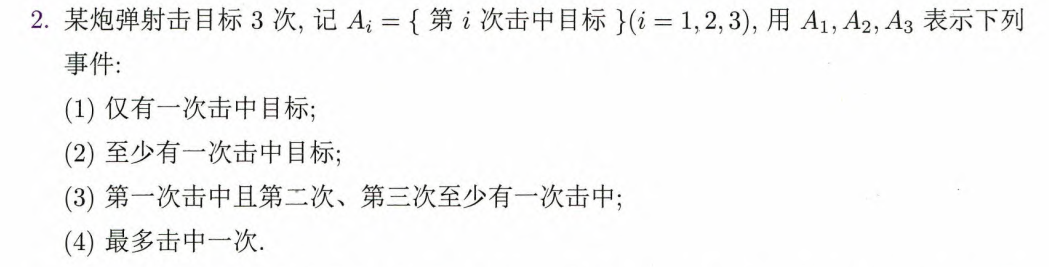#### (1)

$A={A}_{1}{\stackrel{―}{A}}_{2}{\stackrel{―}{A}}_{3}+{\stackrel{―}{A}}_{1}{A}_{2}{\stackrel{―}{A}}_{3}+{\stackrel{―}{A}}_{1}{\stackrel{―}{A}}_{2}{A}_{3}$

#### (2)

$A$$A$ 是“三次均未击中目标”的对立事件，即

$A=\stackrel{―}{{\stackrel{―}{A}}_{1}{\stackrel{―}{A}}_{2}{\stackrel{―}{A}}_{3}}={A}_{1}\cup {A}_{2}\cup {A}_{3}$

#### (3)

“第一次击中”为 ${A}_{1}$$A_1$，而“第二次、第三次至少有一次击中”（记为${A}^{\prime }$$A'$）是“第二次、第三次均未击中”的对立事件，即

${A}^{\prime }=\stackrel{―}{{\stackrel{―}{A}}_{2}{\stackrel{―}{A}}_{3}}$

从而

$A={A}_{1}\cdot {A}^{\prime }={A}_{1}\stackrel{―}{{\stackrel{―}{A}}_{2}{\stackrel{―}{A}}_{3}}={A}_{1}\left({A}_{2}\cup {A}_{3}\right)$

#### (4)

“最多击中一次”是“三次均未击中目标”和“仅有一次击中目标”的和事件，即

$A={\stackrel{―}{A}}_{1}{\stackrel{―}{A}}_{2}{\stackrel{―}{A}}_{3}+{A}_{1}{\stackrel{―}{A}}_{2}{\stackrel{―}{A}}_{3}+{\stackrel{―}{A}}_{1}{A}_{2}{\stackrel{―}{A}}_{3}+{\stackrel{―}{A}}_{1}{\stackrel{―}{A}}_{2}{A}_{3}$

### T1.8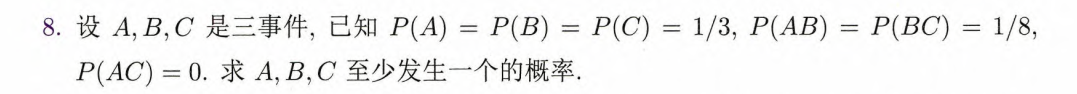所求事件为 $A\cup B\cup C$$A\cup B\cup C$. 由容斥原理（inclusive-exclusive principle），有

$\begin{array}{c}P\left(A\cup B\cup C\right)\\ =P\left(A\right)+P\left(B\right)+P\left(C\right)-P\left(AB\right)-P\left(AC\right)-P\left(BC\right)+P\left(ABC\right)\\ =1/3+1/3+1/3-1/8-0-1/8+0\\ =3/4\end{array}$

### T1.12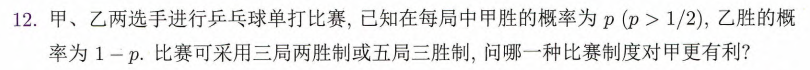$A$$A$ 为“甲胜利”.

考虑三局两胜制，则 $A$$A$ 相当于“连胜两局“与”前两局中甲乙各胜一局且第三局甲胜”的和事件，记为 ${A}_{1}$$A_1$，则

$P\left({A}_{1}\right)={p}^{2}+\left(\genfrac{}{}{0}{}{2}{1}\right)p\left(1-p\right)\cdot p={p}^{2}+2{p}^{2}\left(1-p\right)=-2{p}^{3}+3{p}^{2}$

考虑五局三胜制，则 $A$$A$ 相当于"甲连胜三局"、“前三局甲胜两局乙胜一局且第四局甲胜”与“前四局中甲乙各胜两局且第五局甲胜”，记为 ${A}_{2}$$A_2$，则

$\begin{array}{rl}P\left({A}_{2}\right)& ={p}^{3}+\left(\genfrac{}{}{0}{}{3}{2}\right){p}^{2}\left(1-p\right)\cdot p+\left(\genfrac{}{}{0}{}{4}{2}\right){p}^{2}\left(1-p{\right)}^{2}\cdot p\\ & ={p}^{3}+3{p}^{3}\left(1-p\right)+6{p}^{3}\left({p}^{2}-2p+1\right)\\ & ={p}^{3}+3{p}^{3}-3{p}^{4}+6{p}^{5}-12{p}^{4}+6{p}^{3}\\ & =6{p}^{5}-15{p}^{4}+10{p}^{3}\end{array}$

$\begin{array}{rl}f\left(p\right)& =P\left({A}_{1}\right)-P\left({A}_{2}\right)\\ & =-2{p}^{3}+3{p}^{2}-6{p}^{5}+15{p}^{4}-10{p}^{3}\\ & =-6{p}^{5}+15{p}^{4}-12{p}^{3}+3{p}^{2}\\ & =3{p}^{2}\left(-2{p}^{3}+5{p}^{2}-4p+1\right)\end{array}$

考虑 $g\left(p\right)=-2{p}^{3}+5{p}^{2}-4p+1$$g(p)=-2p^3+5p^2-4p+1$，则

${g}^{\prime }\left(p\right)=-6{p}^{2}+10p-4=-6\left(p-\frac{2}{3}\right)\left(p-1\right)$

从而 $g\left(p\right)$$g(p)$$\left(\frac{1}{2},\frac{2}{3}\right)$$\left(\dfrac12,\dfrac23\right)$ 单调减，在 $\left(\frac{2}{3},1\right)$$\left(\dfrac23,1\right)$ 单调增，又因为 $g\left(\frac{1}{2}\right)=g\left(1\right)=0$$g\left(\dfrac12\right)=g(1)=0$，在 $\left(\frac{1}{2},1\right)$$\left(\dfrac12,1\right)$ 上有 $g\left(p\right)<0$$g(p)<0$，从而 $f\left(p\right)<0$$f(p)<0$，即

$P\left({A}_{1}\right)

即在 $p>\frac{1}{2}$$p>\dfrac12$ 时，五局三胜制更有利.

### T1.13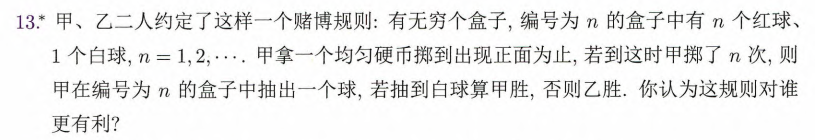${A}_{n}$$A_n$ 为“甲掷了 $n$$n$ 次硬币后出现正面”，则 $P\left(A\right)={\left(1-\frac{1}{2}\right)}^{n-1}\cdot \frac{1}{2}={\left(\frac{1}{2}\right)}^{n}$$P(A) =\left(1-\dfrac12\right)^{n-1}\cdot\dfrac12=\left(\dfrac12\right)^n$

${B}_{n}$$B_n$ 为“甲从第 $n$$n$ 个盒子中抽到白球”，则 $P(B)=\dfrac{1}{n+1}\leqslant\dfrac12\left(n\geqslant1,当且仅当\ n=1\ 时取等\right)$

${C}_{n}$$C_n$ 为“甲掷了 $n$$n$ 次硬币后出现正面并胜利”，则

$P\left({C}_{n}\right)=P\left({A}_{n}{B}_{n}\right)=P\left({A}_{n}\right)P\left({B}_{n}\right)={\left(\frac{1}{2}\right)}^{n}\cdot \frac{1}{n+1}⩽{\left(\frac{1}{2}\right)}^{n}\cdot \frac{1}{2}={\left(\frac{1}{2}\right)}^{n+1}$

$C$$C$ 为“甲胜利”，则

$P\left(C\right)=P\left(\sum _{n=1}^{\mathrm{\infty }}{C}_{n}\right)⩽\sum _{n=1}^{\mathrm{\infty }}P\left({C}_{n}\right)⩽\underset{n\to \mathrm{\infty }}{lim}\left(\sum _{k=1}^{n}{\left(\frac{1}{2}\right)}^{k+1}\right)=\underset{n\to \mathrm{\infty }}{lim}\left(\frac{\frac{1}{4}\left(1-{\left(\frac{1}{2}\right)}^{n+1}\right)}{1-\frac{1}{2}}\right)=\frac{1}{2}$

事实上，有 $P\left(C\right)<\frac{1}{2}$$P(C)<\dfrac12$. 综上，这规则对乙更有利.

### T1.17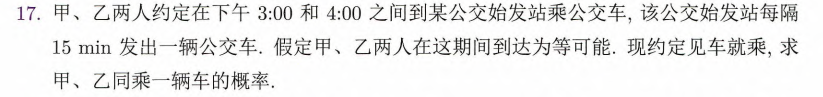设下午 $3:00$$3:00$ 已经发出第一辆公交车，则给定时间内的发车时间为下午 $3:15$$3:15$$3:30$$3:30$$3:45$$3:45$$4:00$$4:00$.

以下午 $3:00$$3:00$ 为基准时间，设甲、乙的到达时间分别为 $x$$x$$y$$y$$0）.

${A}_{1}$$A_1$ 为“甲、乙同乘第一辆车”，则

同理，

易知

$A$$A$ 为“甲、乙同乘一辆车”，则

$P\left(A\right)=P\left(\sum _{i=1}^{4}{A}_{i}\right)=\sum _{i=1}^{4}P\left({A}_{i}\right)=\frac{1}{4}$

### T1.20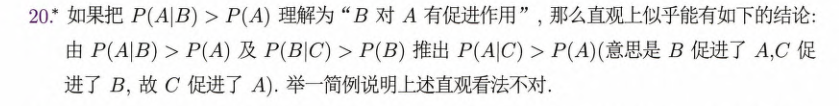考虑掷一六面质地均匀骰子. 设 $A=\{掷出\ 2\ 的倍数\}$$B=\{掷出\ 6\}$$C=\{掷出\ 3\ 的倍数\}$，则

$P\left(A\right)=\frac{1}{3},P\left(B\right)=\frac{1}{6},P\left(C\right)=\frac{1}{2},$
$P\left(AB\right)=P\left(B\right)=\frac{1}{6},P\left(BC\right)=P\left(B\right)=\frac{1}{6},P\left(AC\right)=\frac{1}{6},$
$P\left(A|B\right)=\frac{P\left(AB\right)}{P\left(B\right)}=1,P\left(B|C\right)=\frac{P\left(BC\right)}{P\left(C\right)}=\frac{1}{3},P\left(A|C\right)=\frac{P\left(AC\right)}{P\left(C\right)}=\frac{1}{3}$

$P\left(A|B\right)>P\left(A\right)$$P(A|B)>P(A)$$P\left(B|C\right)>P\left(B\right)$$P(B|C)>P(B)$，但有 $P\left(A|C\right)=P\left(A\right)$$P(A|C)=P(A)$.

### T1.23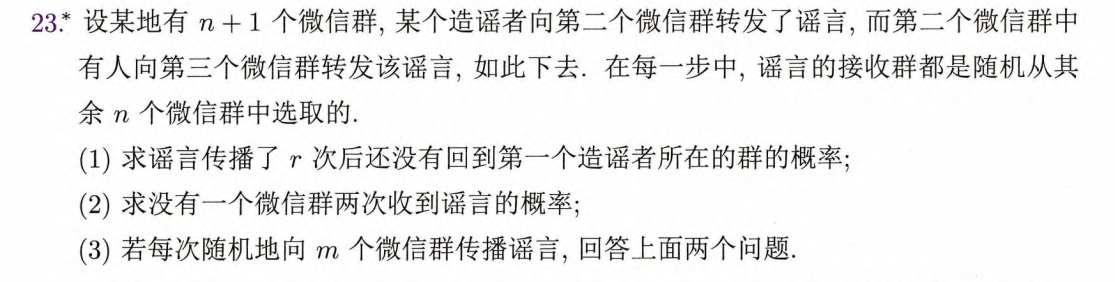#### (1)

${A}_{r}$$A_r$ 为所求事件，则

$P\left({A}_{r}\right)={\left(\frac{n-1}{n}\right)}^{r}$

#### (2)

设谣言传播了 $r$$r$ 次，则：

• $r⩽n$$r\leqslant n$ 时，

$P\left({B}_{r}\right)=\frac{n}{n}\cdot \frac{n-1}{n}\cdot \frac{n-2}{n}\cdots \frac{n-r+1}{n}=\frac{{\mathrm{A}}_{n}^{r}}{{n}^{r}}$
• $r>n$$r > n$ 时，显然有 $P\left({B}_{r}\right)=0$$P(B_r)=0$.

#### (3)

##### (3.1)

${A}_{r}^{\prime }$$A_r'$ 为所求事件，则

$P\left({A}_{r}^{\prime }\right)={\left(\frac{n-1}{n}\right)}^{mr}$
##### (3.2)

设谣言传播了 $r$$r$ 次，则

• $mr⩽n$$mr\leqslant n$ 时，

$\begin{array}{c}\begin{array}{rl}P\left({B}_{r}^{\prime }\right)& =\left(\frac{n}{n}\cdot \frac{n-1}{n}\cdots \frac{n-m+1}{n}\right)\cdot \left(\frac{n-m}{n}\cdot \frac{n-m-1}{n}\cdots \frac{n-2m+1}{n}\right)\cdots \left(\frac{n-\left(r-1\right)m}{n}\cdot \frac{n-\left(r-1\right)m-1}{n}\cdots \frac{n-rm+1}{n}\right)\\ & =\frac{n\left(n-1\right)\cdots \left(n-mr+1\right)}{{n}^{mr}}\\ & =\frac{{\mathrm{A}}_{n}^{mr}}{{n}^{mr}}\end{array}\end{array}$
• $mr>n$$mr>n$ 时，显然有 $P\left({B}_{r}^{\prime }\right)=0$$P(B_r')=0$.

### T1.24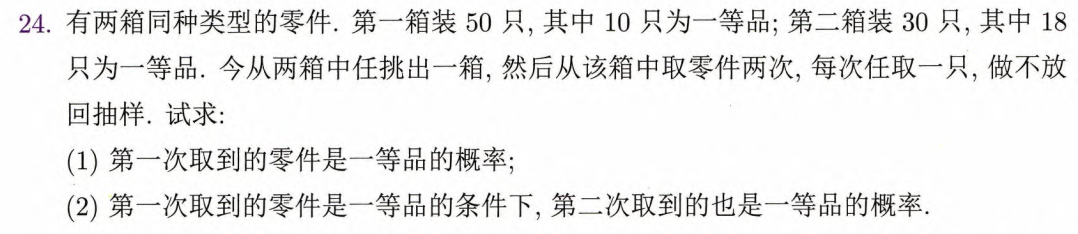#### (1)

考虑从第一箱中取，则第一次取取到一等品的概率为 $\frac{10}{50}$$\dfrac{10}{50}$

考虑从第二箱中取，则第一次取取到一等品的概率为 $\frac{18}{30}$$\dfrac{18}{30}$.

$A=\left\{\text{第}\text{一}\text{次}\text{取}\text{到}\text{的}\text{零}\text{件}\text{是}\text{一}\text{等}\text{品}\right\}$$A=\{第一次取到的零件是一等品\}$，则其概率为

$P\left(A\right)=\frac{1}{2}\cdot \frac{10}{50}+\frac{1}{2}\cdot \frac{18}{30}=\frac{2}{5}$

#### (2)

$B=\left\{\text{第}\text{二}\text{次}\text{取}\text{到}\text{的}\text{零}\text{件}\text{是}\text{一}\text{等}\text{品}\right\}$$B=\{第二次取到的零件是一等品\}$，则 $AB=\left\{\text{两}\text{次}\text{取}\text{到}\text{的}\text{零}\text{件}\text{都}\text{是}\text{一}\text{等}\text{品}\right\}$$AB=\{两次取到的零件都是一等品\}$.

考虑从第一箱中取，则两次取到一等品的概率为 $\frac{{\mathrm{C}}_{10}^{2}}{{\mathrm{C}}_{50}^{2}}=\frac{9}{245}$$\dfrac{\mathrm{C}_{10}^2}{\mathrm{C}_{50}^2}=\dfrac{9}{245}$

考虑从第二箱中取，则两次取到一等品的概率为 $\frac{{\mathrm{C}}_{18}^{2}}{{\mathrm{C}}_{30}^{2}}=\frac{51}{145}$$\dfrac{\mathrm{C}_{18}^2}{\mathrm{C}_{30}^2}=\dfrac{51}{145}$.

从而，

$P\left(AB\right)=\frac{1}{2}\cdot \frac{9}{245}+\frac{1}{2}\cdot \frac{51}{145}=\frac{276}{1421}$

所以有

$P\left(B|A\right)=\frac{P\left(AB\right)}{P\left(A\right)}=\frac{276}{1421}\cdot \frac{5}{2}=\frac{690}{1421}\approx 0.486$

### T1.30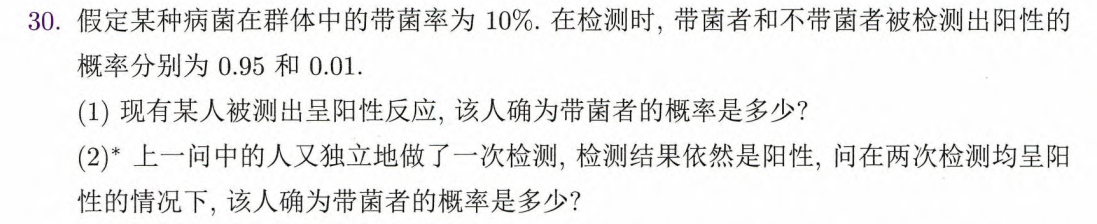Bayes 公式：设 $B$$B$$\stackrel{―}{B}$$\overline B$$\Omega$$\varOmega$ 的一个划分，$P\left(A\right)>0$$P(A)>0$，则

$P\left(B|A\right)=\frac{P\left(AB\right)}{P\left(A\right)}=\frac{P\left(A|B\right)P\left(B\right)}{P\left(A|B\right)P\left(B\right)+P\left(A|\stackrel{―}{B}\right)P\left(\stackrel{―}{B}\right)}$

$A=\left\{\text{测}\text{出}\text{阳}\text{性}\right\}$$A=\{测出阳性\}$$B=\left\{\text{带}\text{菌}\right\}$$B=\{带菌\}$$\stackrel{―}{B}=\left\{\text{不}\text{带}\text{菌}\right\}$$\overline B=\{不带菌\}$. 则 $P\left(B\right)=0.1$$P(B)=0.1$$P\left(\stackrel{―}{B}\right)=0.9$$P(\overline B)=0.9$$P\left(A|B\right)=0.95$$P(A|B)=0.95$$P\left(A|\stackrel{―}{B}\right)=0.01$$P(A|\overline B)=0.01$.

#### (1)

$P\left(B|A\right)=\frac{P\left(A|B\right)P\left(B\right)}{P\left(A|B\right)P\left(B\right)+P\left(A|\stackrel{―}{B}\right)P\left(\stackrel{―}{B}\right)}=\frac{0.95×0.1}{0.95×0.1+0.01×0.9}=\frac{95}{104}\approx 0.913$

#### (2)

$A_i=\{第\ i\ 次测出阳性\},\ i=1,\ 2.$ ${A}^{\prime }={A}_{1}{A}_{2}=\left\{\text{两}\text{次}\text{均}\text{测}\text{出}\text{阳}\text{性}\right\}$$A'=A_1A_2=\{两次均测出阳性\}$，则 $P(A_i|B)=0.95,P(A_i|\overline B)=0.01,\ i=1,\ 2$.

$P\left({A}^{\prime }|B\right)=P\left({A}_{1}{A}_{2}|B\right)=P\left({A}_{1}|B\right)P\left({A}_{2}|B\right)={0.95}^{2}$$P(A'|B)=P(A_1A_2|B)=P(A_1|B)P(A_2|B)=0.95^2$$P\left({A}^{\prime }|\stackrel{―}{B}\right)=P\left({A}_{1}{A}_{2}|\stackrel{―}{B}\right)=P\left({A}_{1}|\stackrel{―}{B}\right)P\left({A}_{2}|\stackrel{―}{B}\right)={0.01}^{2}$$P(A'|\overline B)=P(A_1A_2|\overline B)=P(A_1|\overline B)P(A_2|\overline B)=0.01^2$.

$P\left(B|{A}^{\prime }\right)=\frac{P\left({A}^{\prime }|B\right)P\left(B\right)}{P\left({A}^{\prime }|B\right)P\left(B\right)+P\left({A}^{\prime }|\stackrel{―}{B}\right)P\left(\stackrel{―}{B}\right)}=\frac{{0.95}^{2}×0.1}{{0.95}^{2}×0.1+{0.01}^{2}×0.9}=\frac{9025}{9034}\approx 0.999$

### T1.33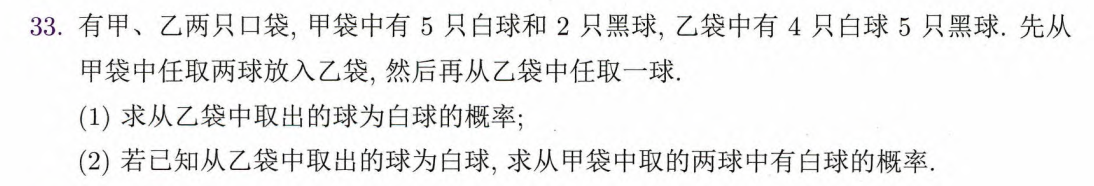$A_i=\{从甲袋中取\ i\ 个白球和 (2-i)个黑球放入乙袋\},\ i=0,\ 1,\ 2$$B=\left\{\text{从}\text{乙}\text{袋}\text{中}\text{取}\text{出}\text{白}\text{球}\right\}$$B=\{从乙袋中取出白球\}$，则 ${A}_{i}$$A_i$ 是样本空间 $\Omega$$\varOmega$ 的一个划分.

$P\left({A}_{0}\right)=\frac{{\mathrm{C}}_{2}^{2}}{{\mathrm{C}}_{7}^{2}}=\frac{1}{21}$$P(A_0)=\dfrac{\mathrm{C}_2^2}{\mathrm{C}_7^2}=\dfrac{1}{21}$$P\left({A}_{1}\right)=\frac{{\mathrm{C}}_{5}^{1}{\mathrm{C}}_{2}^{1}}{{\mathrm{C}}_{7}^{2}}=\frac{10}{21}$$P(A_1)=\dfrac{\mathrm{C}_5^1\mathrm{C}_2^1}{\mathrm{C}_7^2}=\dfrac{10}{21}$$P\left({A}_{2}\right)=\frac{{\mathrm{C}}_{5}^{2}}{{\mathrm{C}}_{7}^{2}}=\frac{10}{21}$$P(A_2)=\dfrac{\mathrm{C}_5^2}{\mathrm{C}_7^2}=\dfrac{10}{21}$

$P\left(B|{A}_{0}\right)=\frac{4}{11}$$P(B|A_0)=\dfrac{4}{11}$$P\left(B|{A}_{1}\right)=\frac{5}{11}$$P(B|A_1)=\dfrac{5}{11}$$P\left(B|{A}_{2}\right)=\frac{6}{11}$$P(B|A_2)=\dfrac{6}{11}$.

#### (1)

考察全概率公式.

$P\left(B\right)=\sum _{i=0}^{2}P\left(B|{A}_{i}\right)P\left({A}_{i}\right)=\frac{4}{11}\cdot \frac{1}{21}+\frac{5}{11}\cdot \frac{10}{21}+\frac{6}{11}\cdot \frac{10}{21}=\frac{38}{77}$

#### (2)

考察 Bayes 公式.

首先计算“已知从乙袋中取出的球为白球，从甲袋中取的两球中无白球”的概率，也就是 $P\left({A}_{0}|B\right)$$P(A_0|B)$.

$P\left({A}_{0}|B\right)=\frac{P\left(B{A}_{0}\right)}{P\left(B\right)}=\frac{P\left(B|{A}_{0}\right)P\left({A}_{0}\right)}{\sum _{i=0}^{2}P\left(B|{A}_{i}\right)P\left({A}_{i}\right)}=\frac{\frac{4}{11}\cdot \frac{1}{21}}{\frac{38}{77}}=\frac{2}{57}$

所求概率为其对立事件的概率，则

$P=P\left({\stackrel{―}{A}}_{0}|B\right)=1-P\left({A}_{0}|B\right)=1-\frac{2}{57}=\frac{55}{57}$

摆了

## HW3

### T2.22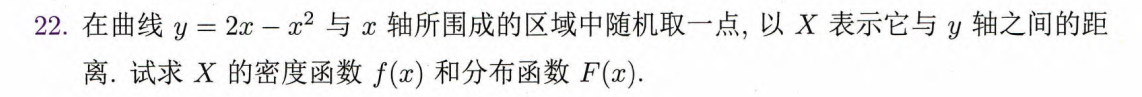$\begin{array}{c}F\left(x\right)=-\frac{{x}^{3}}{4}+\frac{3}{4}{x}^{2},0⩽x⩽2,\\ f\left(x\right)={F}^{\prime }\left(x\right)=-\frac{3}{4}{x}^{2}+\frac{3}{2}x,0⩽x<2.\end{array}$

### T2.27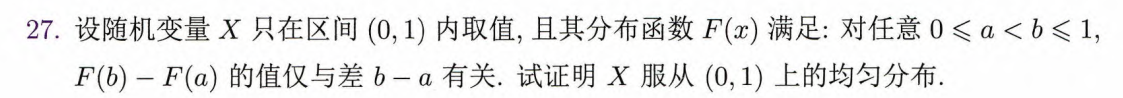固定 $a$$a$，令 $b=a+t$$b=a+t$，则 $0\leqslant a <1,\ 0.

由题意，设 $F\left(a+t\right)-F\left(a\right)=g\left(t\right)$$F(a+t)-F(a)=g(t)$，则

$\frac{F\left(a+t\right)-F\left(a\right)}{t}=\frac{g\left(t\right)}{t}$

$t\to 0$$t\to0$，则

$\underset{t\to 0}{lim}\frac{g\left(t\right)}{t}={F}^{\prime }\left(a\right)=f\left(a\right).$

$\underset{t\to 0}{lim}g\left(t\right)=0$$\displaystyle\lim_{t\to0}g(t)=0$$\underset{t\to 0}{lim}\frac{g\left(t\right)}{t}=c$$\displaystyle\lim_{t\to0}\dfrac{g(t)}t=c$.

这个结论与 $a$$a$ 的取值无关，故

${F}^{\prime }\left(a\right)=f\left(a\right)=c,0

又因为

计算得 $c=1$$c=1$，即 $f\left(x\right)=1,0$f(x)=1,0. 同时，

易知 $X$$X$ 服从 $\left(0,1\right)$$(0,1)$ 上的均匀分布.

### T2.37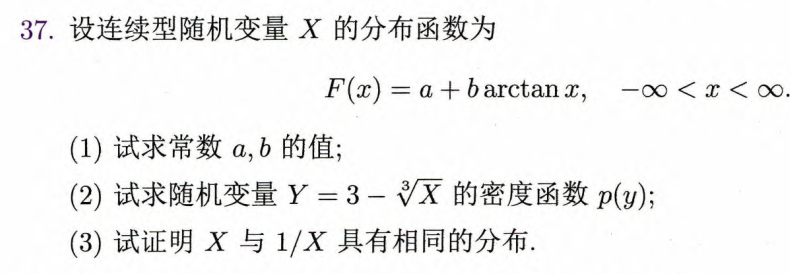(3)

$Y=1/X$$Y=1/X$. $g\left(x\right)=1/x$$g(x)=1/x$$\left(-\mathrm{\infty },0\right),\left(0,+\mathrm{\infty }\right)$$(-\infty,0),(0,+\infty)$ 分别单调减.

${I}_{1}=\left(-\mathrm{\infty },0\right)$$I_1=(-\infty,0)$ 上，$g\left(x\right)=1/x$$g(x)=1/x$ 有唯一反函数 ${h}_{1}\left(y\right)=1/y$$h_1(y)=1/y$，其导函数 ${h}_{1}^{\prime }\left(y\right)=-1/{y}^{2}$$h'_1(y)=-1/y^2$ 连续，则

$f\left({h}_{1}\left(y\right)\right)|{h}_{1}^{\prime }\left(y\right)|{I}_{1}=\frac{1}{\pi \left(1+\left(1/y{\right)}^{2}\right)}\cdot |-\frac{1}{{y}^{2}}|\cdot {I}_{\left(-\mathrm{\infty },0\right)}=\frac{1}{\pi \left(1+{y}^{2}\right)}{I}_{\left(-\mathrm{\infty },0\right)};$

${I}_{2}=\left(0,+\mathrm{\infty }\right)$$I_2=(0,+\infty)$ 上，同理可得

$f\left({h}_{2}\left(y\right)\right)|{h}_{2}^{\prime }\left(y\right)|{I}_{2}=\frac{1}{\pi \left(1+{y}^{2}\right)}{I}_{\left(0,+\mathrm{\infty }\right)}.$

此时由密度变换公式，